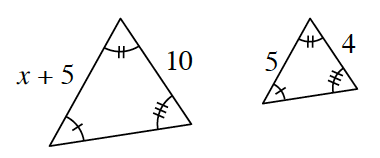Home > INT2 > Chapter 4 > Lesson 4.1.3 > Problem4-30

4-30.

Examine the triangles below. Solve for $x$. Homework Help ✎Decide if the triangles are similar. Review the Math Notes boxes in Lessons 3.2.1 and 3.2.5 for help.

Decide which sides of the triangles correspond.

Write a proportion and solve for $x$.